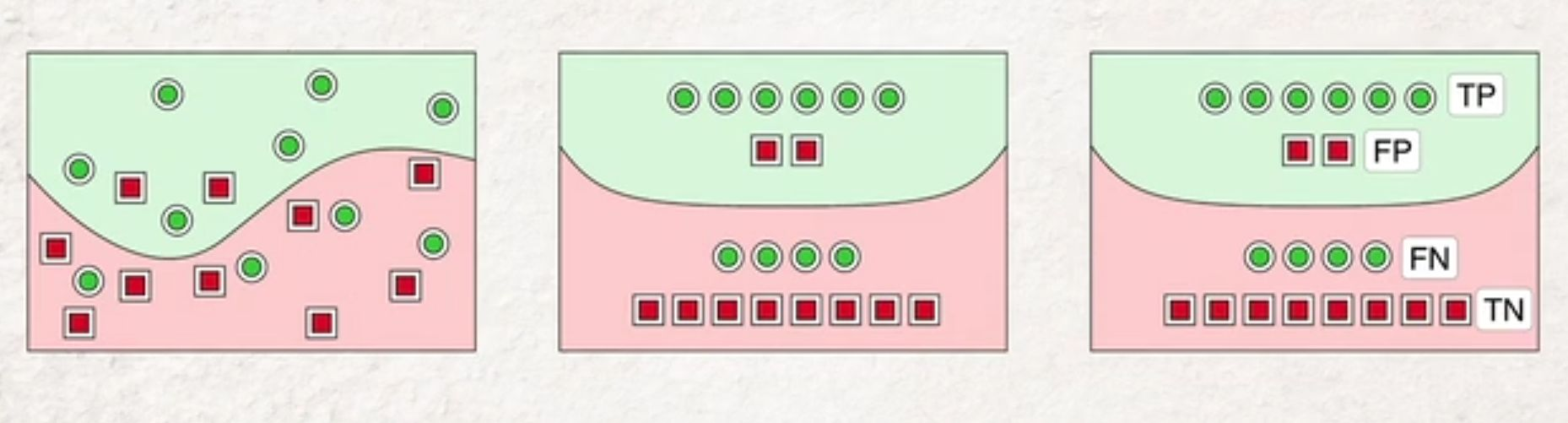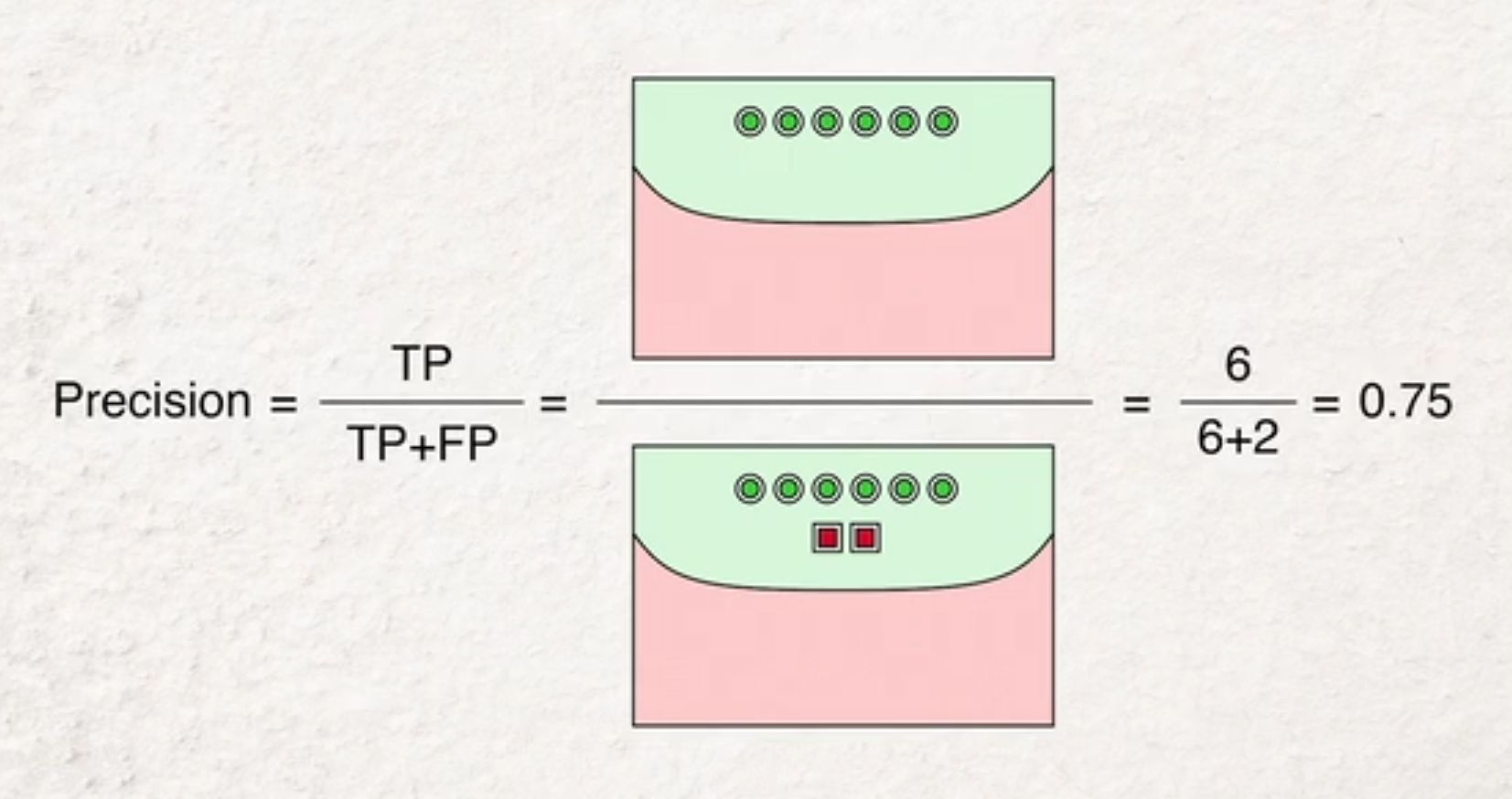Show Question
Math and science::INF ML AI

# Accuracy, precision, recall\begin{aligned} \text{accuracy} &= \frac{TP + TN}{TP + TN + FP + FN} \\ \text{precision} &= \frac{TP}{TP + FP} \\ \text{recall} &= \frac{TP}{TP + FN} \\ \end{aligned}

### Formulated in words

accuracy = $$\frac{ \text{correct} }{ \text{incorrect} }$$

precision = given a positive result, how likely is the true state to be positive; the reliability of a positive result.

recall = how many of the true positives are recoreded as positive. Have we missed many positives?

### Pathalogical and benign examples

Are false positives dangerous? Are false negatives dangerous? Poor precision affords many false positives. Poor recall affords many false negatives. If the true/false meaning of any test is reversed, false positives become false negatives and vice versa.

• Poor precision is pathalogical: COVID19 testing where positive represents being free of the virus.
• Poor precision is benign: COVID19 testing, where a positive represents having the virus.
• Poor recall is pathalogical: COVID19 testing, where a positive represents having the virus.
• Poor recall is benign: COVID19 testing where positive represents being free of the virus.